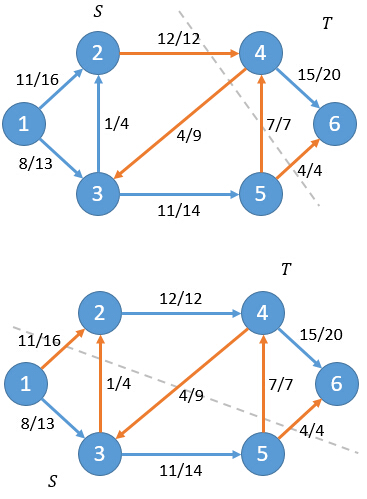# hihocoder 网络流二·最大流最小割定理

### 网络流二·最大流最小割定理

#### 描述

f(S,T) = Σf(u,v) | u∈S,v∈T净流f = f(2,4)+f(3,4)+f(3,5) = 12+(-4)+11 = 19

C(S,T) = Σc(u,v) | u∈S,v∈T

c(2,4)+c(3,5)=12+11=23f(S,T) = f(S,V) - f(S,S)

f(S,T) = f(S,V)

f(S,T) = f(s,V) + f(S-s,V)

f(S,T) = f(s,V)

f(S,T) = f(s,V) = f

对于一个网络流图G=(V,E)，其中有源点s和汇点t，那么下面三个条件是等价的：
1. 流f是图G的最大流
2. 残留网络Gf不存在增广路
3. 对于G的某一个割(S,T)，此时f = C(S,T)

我们利用反证法，假设流f是图G的最大流，但是残留网络中还存在有增广路p，其流量为fp。则我们有流f'=f+fp>f。这与f是最大流产生矛盾。

假设残留网络Gf不存在增广路，所以在残留网络Gf中不存在路径从s到达t。我们定义S集合为：当前残留网络中s能够到达的点。同时定义T=V-S。

由于f的上界为最小割，当f到达割的容量时，显然就已经到达最大值，因此f为最大流。

#### 输出

6 7
1 2 3
1 3 5
2 4 1
3 4 2
3 5 3
4 6 4
5 6 2

5 4
1 2 3 5分析：最小割最大流，dicnic；代码：
#include <iostream>
#include <cstdio>
#include <cstdlib>
#include <cmath>
#include <algorithm>
#include <climits>
#include <cstring>
#include <string>
#include <set>
#include <map>
#include <queue>
#include <stack>
#include <vector>
#include <list>
#define rep(i,m,n) for(i=m;i<=n;i++)
#define rsp(it,s) for(set<int>::iterator it=s.begin();it!=s.end();it++)
#define mod 1000000007
#define inf 0x3f3f3f3f
#define vi vector<int>
#define pb push_back
#define mp make_pair
#define fi first
#define se second
#define ll long long
#define pi acos(-1.0)
#define pii pair<int,int>
#define Lson L, mid, rt<<1
#define Rson mid+1, R, rt<<1|1
const int maxn=5e2+10;
using namespace std;
ll gcd(ll p,ll q){return q==0?p:gcd(q,p%q);}
ll qpow(ll p,ll q){ll f=1;while(q){if(q&1)f=f*p;p=p*p;q>>=1;}return f;}
int n,m,k,t,h[maxn],tot,vis[maxn],s,cur[maxn];
bool flag;
set<int>ans;
struct node
{
int to,nxt,cap,flow;
}e[20000<<1];
{
e[tot].to=y;
e[tot].nxt=h[x];
e[tot].cap=z;
h[x]=tot++;
e[tot].to=x;
e[tot].nxt=h[y];
h[y]=tot++;
}
bool bfs()
{
memset(vis,0,sizeof vis);
queue<int>p;
p.push(s);
vis[s]=1;
if(flag)ans.insert(s);
while(!p.empty())
{
int x=p.front();p.pop();
for(int i=h[x];i!=-1;i=e[i].nxt)
{
int to=e[i].to,cap=e[i].cap,flow=e[i].flow;
if(!vis[to]&&cap>flow)
{
vis[to]=vis[x]+1;
p.push(to);
if(flag)ans.insert(to);
}
}
}
return vis[t];
}
int dfs(int x,int a)
{
if(x==t||a==0)return a;
int ans=0,j;
for(int&i=cur[x];i!=-1;i=e[i].nxt)
{
int to=e[i].to,cap=e[i].cap,flow=e[i].flow;
if(vis[to]==vis[x]+1&&(j=dfs(to,min(a,cap-flow)))>0)
{
e[i].flow+=j;
e[i^1].flow-=j;
ans+=j;
a-=j;
if(a==0)break;
}
}
return ans;
}
int max_flow(int s,int t)
{
int flow=0,i;
while(bfs())
{
rep(i,1,n)cur[i]=h[i];
flow+=dfs(s,inf);
}
return flow;
}
int main()
{
int i,j;
memset(h,-1,sizeof h);
scanf("%d%d",&n,&m);
while(m--)
{
int a,b,c;
scanf("%d%d%d",&a,&b,&c);
}